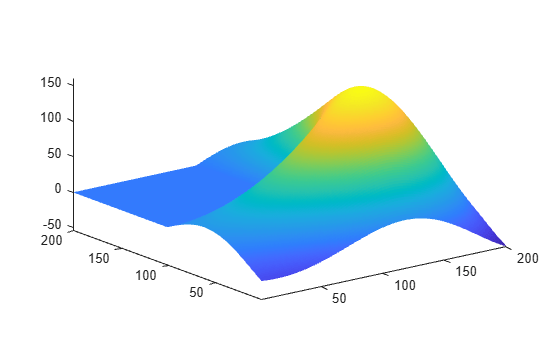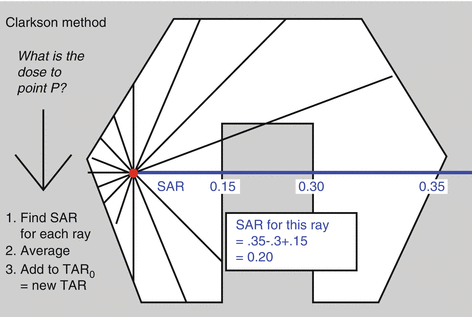matlab source clarkson dose calculationPublish: 2020-May-28,18:05 PM
Visit: 1313
Category:     matlab programming

In this code written in MATLAB programming language, the dose value is calculated using Clarkson algorithm. Clarkson algorithm is a dose calculation algorithm that calculates PDD value using SAR tables.

What is the Clarkson Algorithm?

Clarkson algorithm is a dose calculation algorithm that calculates PDD using SAR table. In this method, we draw segments at the desired point. These sectors are usually equal to 36 sectors.We have a specific radius for each segment, which depends on the point.

The SAR value varies depending on the intended depth and radius. So at the end we have 36 SAR

For 36 SARs, the mean SAR value is obtained.

Using the relationship between SAR and TAR, we calculate the TAR value

TAR = TAR0 + SAR.Mean;

And in the last step using the relation PDD = 100 * TAR * ((f + dm) / (f + d)) ^ 2 / BSF; We get the PDD value at the desired point and the desired depth

What does a writing program do?

In the written program, the dose is calculated using the above algorithm.

How deep can the dose be calculated?

The program is written for a depth of 4 cm. By changing the value of the SARValue table, you can calculate the PDD value for each depth

Tags: